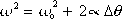omega-squared =  omega-sub-zero-squared    plus    two-alpha times delta-theta

This is one of the constant angular acceleration equations. It's the one with no "t" in it.

omega [radians-per-second] is the angular velocity of the object at time t.
omega-sub-zero [radians-per-second] is the angular velocity of the object at time 0.
alpha [radians-per-seconds-squared] is the angular acceleration.
delta-theta is theta minus theta-sub-zero. It is the angular displacement from time 0 to time t.

Note: "Angular Motion" is synonymous with "Rotational Motion". In the discussion of rotational motion, the words "angular" and "rotational" are interchangeable except in the case of the rotational inertia  I  which is referred to as "rotational inertia" or as the "moment of inertia" but is not referred to as "angular inertia".

Tip: In a constant angular acceleration problem, list the variable names with their values and the sought quantity with a question mark. Use the constant angular acceleration equation that is missing the variable not listed.